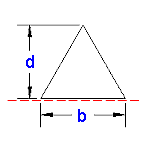Area Moment of Inertia Section Properties : Triangle at Edge Calculator

Area Moment of Inertia Section Properties: Triangle Edge Calculator

Area Moment of Inertia Section Properties of Triangle at Edge Feature Calculator and Equations. This engineering calculator will determine the section modulus for the given cross-section. This engineering data is often used in the design of structural beams or structural flexural members.

 Description Equation Area Moment of Inertia = ISection Modulus = Z = I/yRadius of GyrationA = area 1/2(db) y = Distance to neutral axis dArea Moment of Inertia Section Properties: Triangle Edge Calculator Variables Inputs: Inch (in.) Metric (mm) "b" (Base) = "d" (Height) = Properties: Area Moment of Inertia (Units4) = Section Modulus (Units3) = Radius of Gyration (Units) = Extreme Point (Units) = Area (Units2) =Membership Register | LoginHomeEngineering Book StoreEngineering ForumExcel App. DownloadsOnline Books & ManualsEngineering NewsEngineering VideosEngineering CalculatorsEngineering ToolboxGD&T Training Geometric Dimensioning TolerancingDFM DFA TrainingTraining Online EngineeringAdvertising CenterCopyright Notice